# Principal Components Analysis PCA Principal Components Analysis PCA

• Slides: 39Principal Components Analysis (PCA)Principal Components Analysis (PCA) a technique for finding patterns in data of high dimensionOutline: 1. Eigenvectors and eigenvalues 2. PCA: a) Getting the data b) Centering the data c) Obtaining the covariance matrix d) Performing an eigenvalue decomposition of the covariance matrix e) Choosing components and forming a feature vector f) Deriving the new data set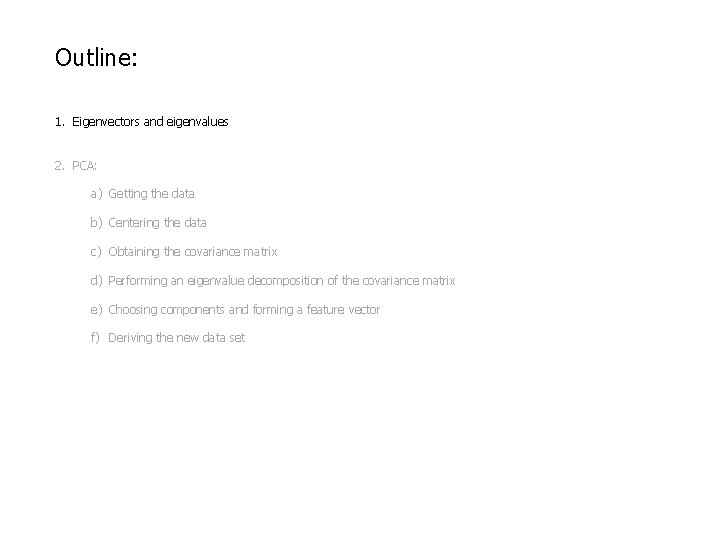Outline: 1. Eigenvectors and eigenvalues 2. PCA: a) Getting the data b) Centering the data c) Obtaining the covariance matrix d) Performing an eigenvalue decomposition of the covariance matrix e) Choosing components and forming a feature vector f) Deriving the new data setEigenvectors & eigenvalues: 2 3 2 1Eigenvectors & eigenvalues: 2 3 2 1 * 1 3Eigenvectors & eigenvalues: 2 3 2 1 * 1 3 = 11 5Eigenvectors & eigenvalues: 2 3 2 1 * * 1 3 3 2 = 11 5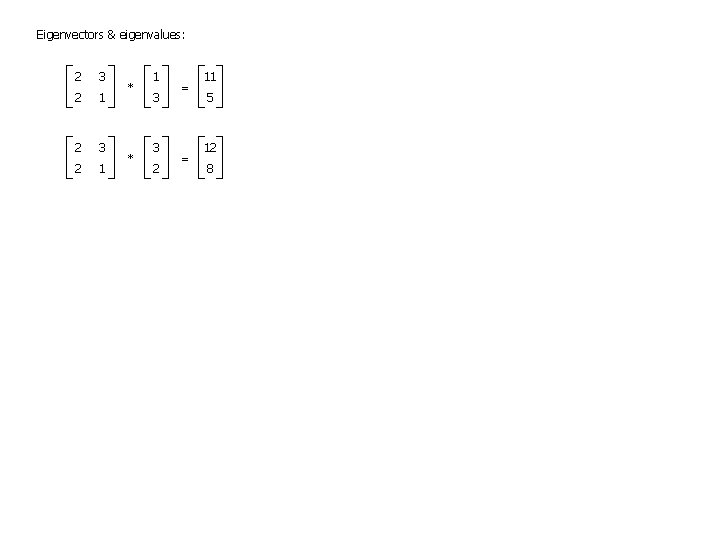Eigenvectors & eigenvalues: 2 3 2 1 * * 1 3 3 2 = = 11 5 12 8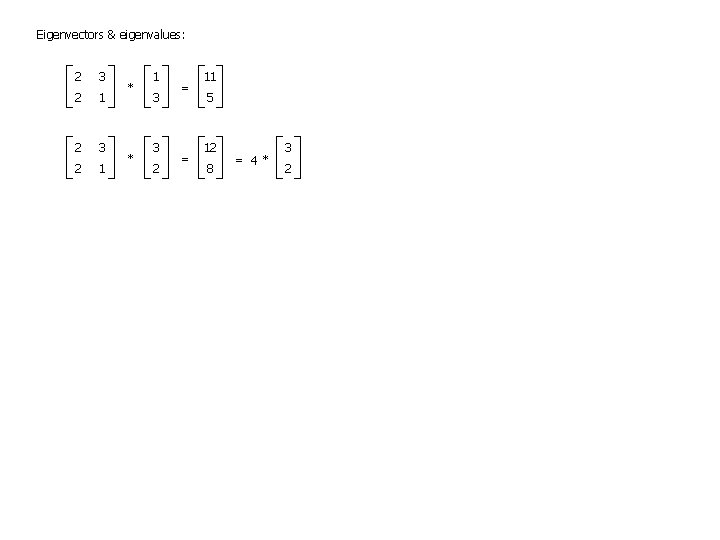Eigenvectors & eigenvalues: 2 3 2 1 * * 1 3 3 2 = = 11 5 12 8 = 4* 3 2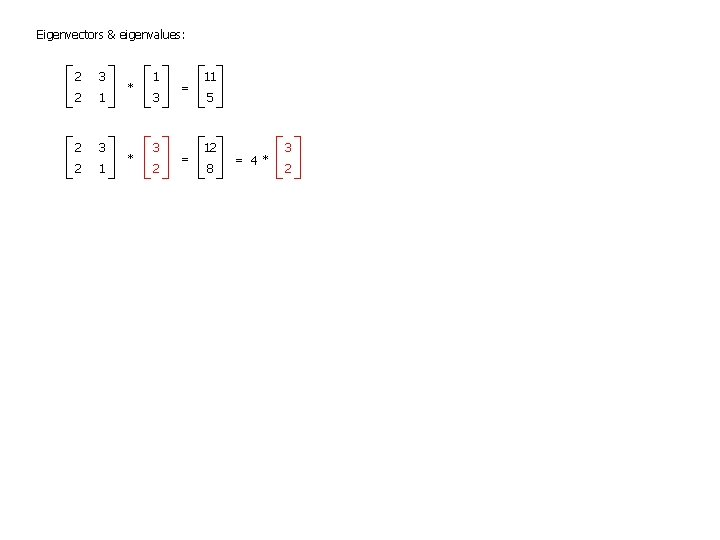Eigenvectors & eigenvalues: 2 3 2 1 * * 1 3 3 2 = = 11 5 12 8 = 4* 3 2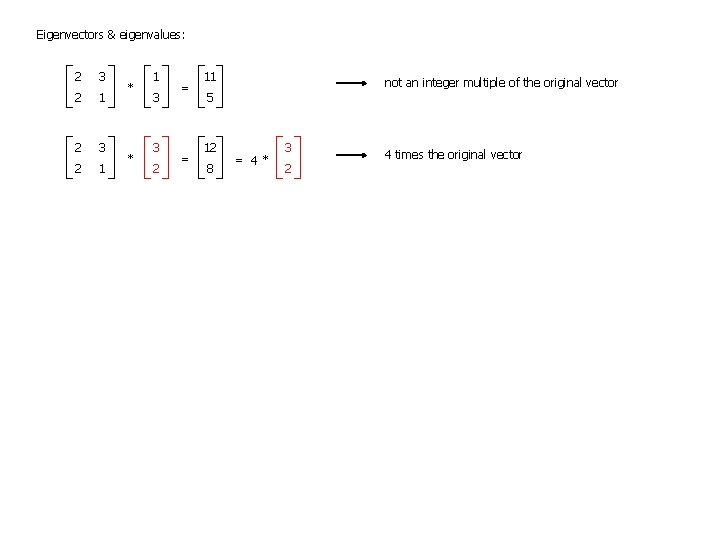Eigenvectors & eigenvalues: 2 3 2 1 * * 1 3 3 2 = = 11 not an integer multiple of the original vector 5 12 8 = 4* 3 2 4 times the original vector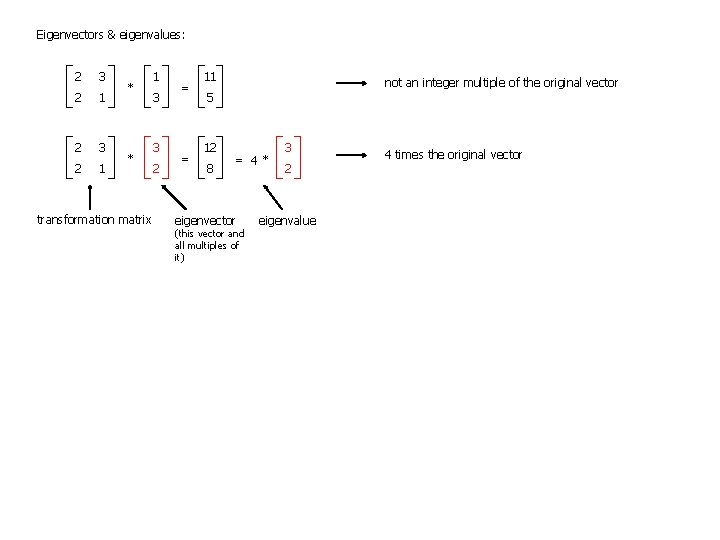Eigenvectors & eigenvalues: 2 3 2 1 * * transformation matrix 1 3 3 2 = = 11 not an integer multiple of the original vector 5 12 8 = 4* eigenvector (this vector and all multiples of it) 3 2 eigenvalue 4 times the original vectorEigenvectors & eigenvalues: 2 3 2 1 * * transformation matrix 1 3 3 2 = = 11 not an integer multiple of the original vector 5 12 8 = 4* eigenvector (this vector and all multiples of it) 8 2 (3, 2) 3 12 3 2 eigenvalue 4 times the original vector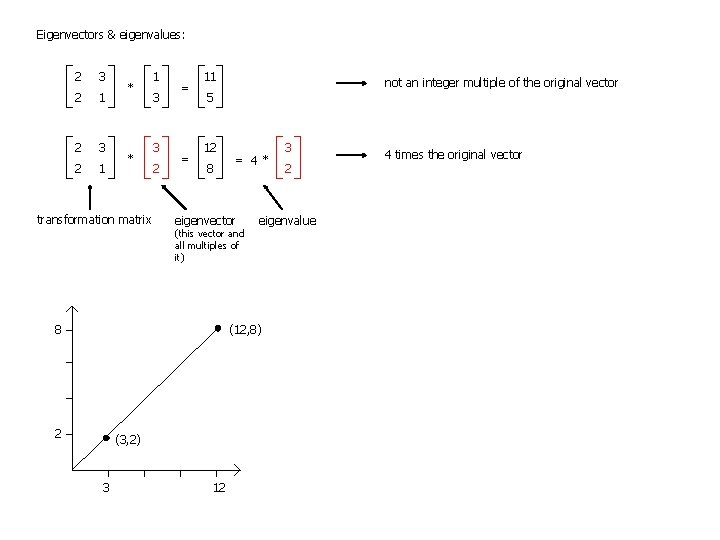Eigenvectors & eigenvalues: 2 3 2 1 * * transformation matrix 1 3 3 2 = = 11 not an integer multiple of the original vector 5 12 8 = 4* eigenvector (this vector and all multiples of it) 8 (3, 2) 3 12 2 eigenvalue (12, 8) 2 3 4 times the original vectorEigenvectors & eigenvalues: 2 3 2 1 * * transformation matrix 1 3 3 2 = = 11 not an integer multiple of the original vector 5 12 8 = 4* eigenvector (this vector and all multiples of it) 8 (3, 2) 3 12 2 4 times the original vector eigenvalue (12, 8) 2 3 Some properties of eigenvectors: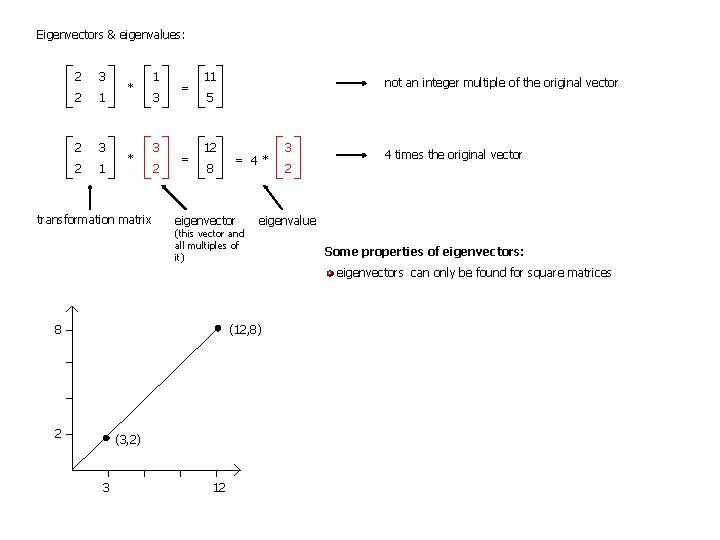Eigenvectors & eigenvalues: 2 3 2 1 * * transformation matrix 1 3 3 2 = = 11 not an integer multiple of the original vector 5 12 8 = 4* eigenvector (this vector and all multiples of it) 3 2 4 times the original vector eigenvalue Some properties of eigenvectors: eigenvectors can only be found for square matrices 8 (12, 8) 2 (3, 2) 3 12Eigenvectors & eigenvalues: 2 3 2 1 * * transformation matrix 1 3 3 2 = = 11 not an integer multiple of the original vector 5 12 8 = 4* eigenvector (this vector and all multiples of it) 3 2 4 times the original vector eigenvalue Some properties of eigenvectors: eigenvectors can only be found for square matrices not every square matrix has eigenvectors 8 (12, 8) 2 (3, 2) 3 12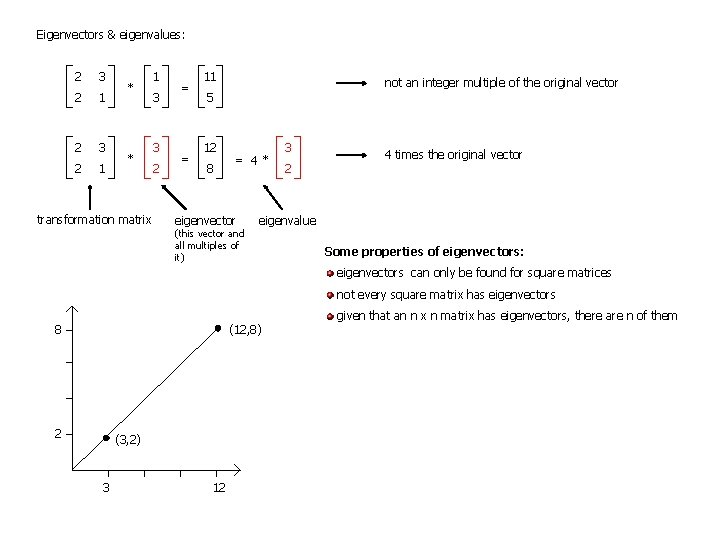Eigenvectors & eigenvalues: 2 3 2 1 * * transformation matrix 1 3 3 2 = = 11 not an integer multiple of the original vector 5 12 8 = 4* eigenvector (this vector and all multiples of it) 3 2 4 times the original vector eigenvalue Some properties of eigenvectors: eigenvectors can only be found for square matrices not every square matrix has eigenvectors 8 (12, 8) 2 (3, 2) 3 12 given that an n x n matrix has eigenvectors, there are n of them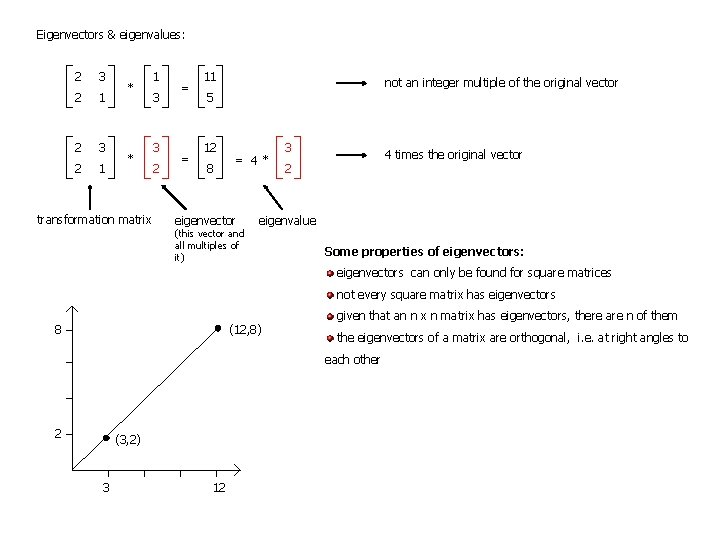Eigenvectors & eigenvalues: 2 3 2 1 * * transformation matrix 1 3 3 2 = = 11 not an integer multiple of the original vector 5 12 8 = 4* eigenvector (this vector and all multiples of it) 3 4 times the original vector 2 eigenvalue Some properties of eigenvectors: eigenvectors can only be found for square matrices not every square matrix has eigenvectors 8 (12, 8) given that an n x n matrix has eigenvectors, there are n of them the eigenvectors of a matrix are orthogonal, i. e. at right angles to each other 2 (3, 2) 3 12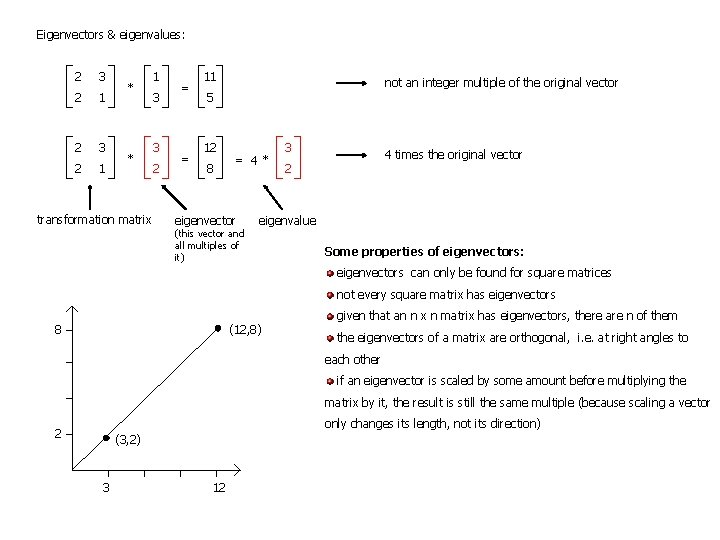Eigenvectors & eigenvalues: 2 3 2 1 * * transformation matrix 1 3 3 2 = = 11 not an integer multiple of the original vector 5 12 8 = 4* eigenvector (this vector and all multiples of it) 3 4 times the original vector 2 eigenvalue Some properties of eigenvectors: eigenvectors can only be found for square matrices not every square matrix has eigenvectors 8 (12, 8) given that an n x n matrix has eigenvectors, there are n of them the eigenvectors of a matrix are orthogonal, i. e. at right angles to each other if an eigenvector is scaled by some amount before multiplying the matrix by it, the result is still the same multiple (because scaling a vector only changes its length, not its direction) 2 (3, 2) 3 12Eigenvectors & eigenvalues: 2 3 2 1 * * transformation matrix 1 3 3 2 = = 11 not an integer multiple of the original vector 5 12 8 = 4* eigenvector (this vector and all multiples of it) 3 4 times the original vector 2 eigenvalue Some properties of eigenvectors: eigenvectors can only be found for square matrices not every square matrix has eigenvectors 8 (12, 8) given that an n x n matrix has eigenvectors, there are n of them the eigenvectors of a matrix are orthogonal, i. e. at right angles to each other if an eigenvector is scaled by some amount before multiplying the matrix by it, the result is still the same multiple (because scaling a vector only changes its length, not its direction) 2 (3, 2) 3 12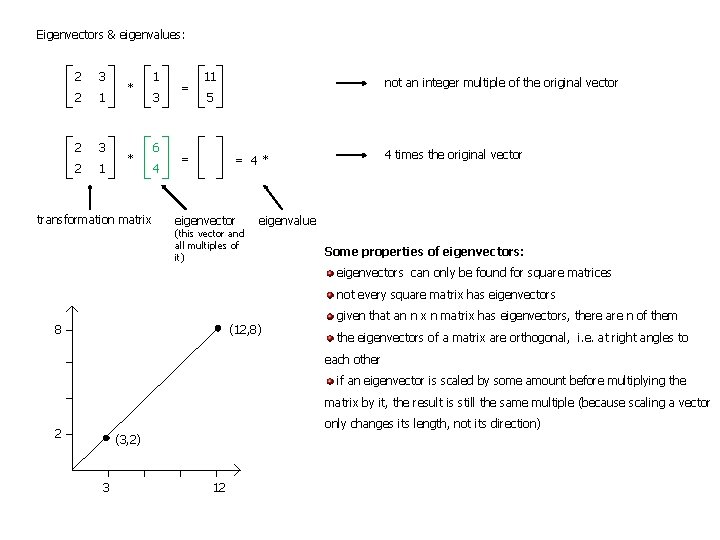Eigenvectors & eigenvalues: 2 3 2 1 * * transformation matrix 1 3 6 4 = 11 not an integer multiple of the original vector 5 = 4 times the original vector = 4* eigenvector (this vector and all multiples of it) eigenvalue Some properties of eigenvectors: eigenvectors can only be found for square matrices not every square matrix has eigenvectors 8 (12, 8) given that an n x n matrix has eigenvectors, there are n of them the eigenvectors of a matrix are orthogonal, i. e. at right angles to each other if an eigenvector is scaled by some amount before multiplying the matrix by it, the result is still the same multiple (because scaling a vector only changes its length, not its direction) 2 (3, 2) 3 12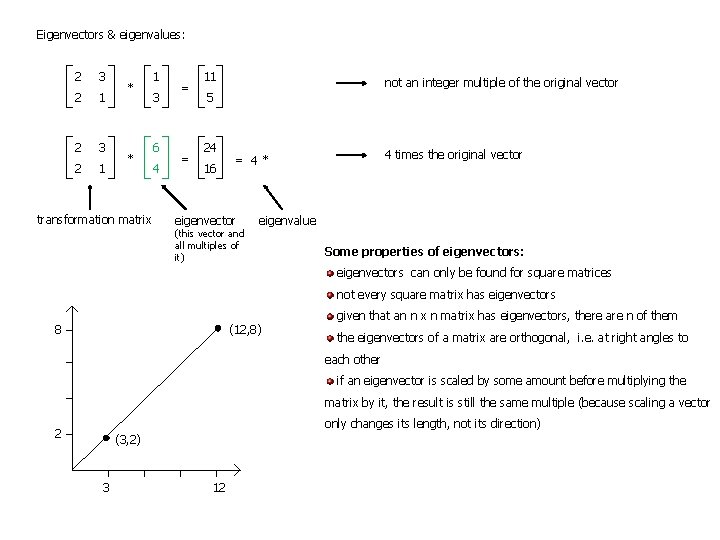Eigenvectors & eigenvalues: 2 3 2 1 * * transformation matrix 1 3 6 4 = = 11 not an integer multiple of the original vector 5 24 16 4 times the original vector = 4* eigenvector (this vector and all multiples of it) eigenvalue Some properties of eigenvectors: eigenvectors can only be found for square matrices not every square matrix has eigenvectors 8 (12, 8) given that an n x n matrix has eigenvectors, there are n of them the eigenvectors of a matrix are orthogonal, i. e. at right angles to each other if an eigenvector is scaled by some amount before multiplying the matrix by it, the result is still the same multiple (because scaling a vector only changes its length, not its direction) 2 (3, 2) 3 12Eigenvectors & eigenvalues: 2 3 2 1 * * transformation matrix 1 3 6 4 = = 11 not an integer multiple of the original vector 5 24 16 = 4* eigenvector (this vector and all multiples of it) 6 4 times the original vector 4 eigenvalue Some properties of eigenvectors: eigenvectors can only be found for square matrices not every square matrix has eigenvectors 8 (12, 8) given that an n x n matrix has eigenvectors, there are n of them the eigenvectors of a matrix are orthogonal, i. e. at right angles to each other if an eigenvector is scaled by some amount before multiplying the matrix by it, the result is still the same multiple (because scaling a vector only changes its length, not its direction) 2 (3, 2) 3 12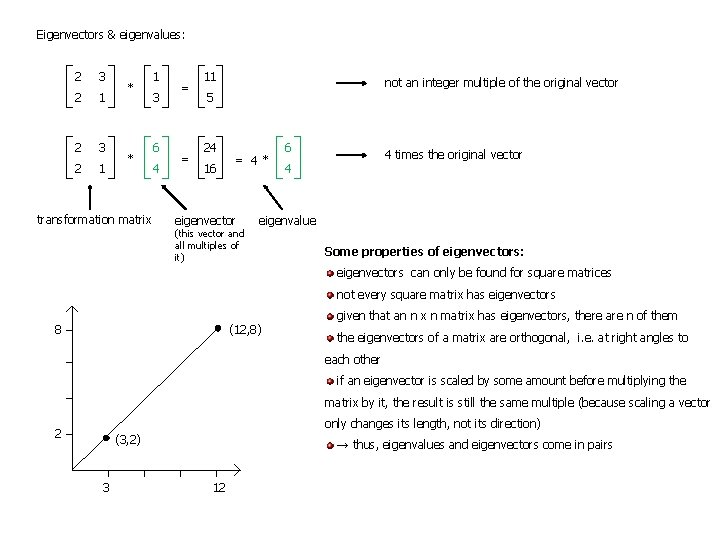Eigenvectors & eigenvalues: 2 3 2 1 * * transformation matrix 1 3 6 4 = = 11 not an integer multiple of the original vector 5 24 16 = 4* eigenvector (this vector and all multiples of it) 6 4 times the original vector 4 eigenvalue Some properties of eigenvectors: eigenvectors can only be found for square matrices not every square matrix has eigenvectors 8 (12, 8) given that an n x n matrix has eigenvectors, there are n of them the eigenvectors of a matrix are orthogonal, i. e. at right angles to each other if an eigenvector is scaled by some amount before multiplying the matrix by it, the result is still the same multiple (because scaling a vector only changes its length, not its direction) 2 (3, 2) 3 → thus, eigenvalues and eigenvectors come in pairs 12Outline: 1. Eigenvectors and eigenvalues 2. PCA: a) Getting the data b) Centering the data c) Obtaining the covariance matrix d) Performing an eigenvalue decomposition of the covariance matrix e) Choosing components and forming a feature vector f) Deriving the new data set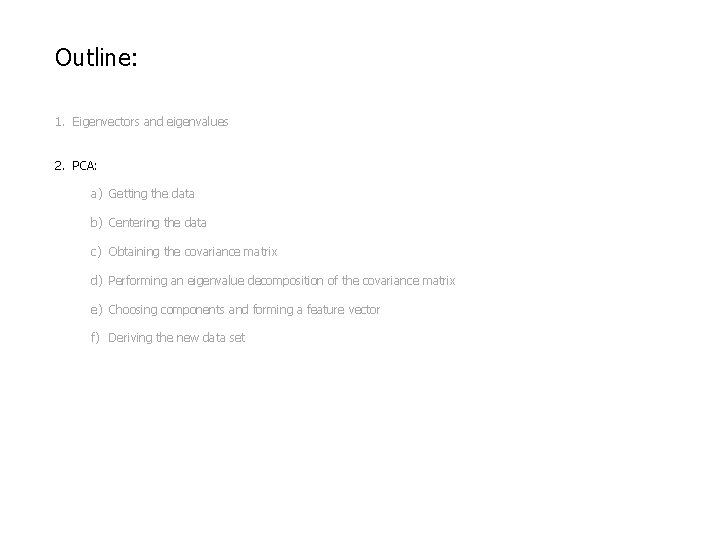Outline: 1. Eigenvectors and eigenvalues 2. PCA: a) Getting the data b) Centering the data c) Obtaining the covariance matrix d) Performing an eigenvalue decomposition of the covariance matrix e) Choosing components and forming a feature vector f) Deriving the new data setPCA - a technique for identifying patterns in data and expressing the data in such a way as to highlight their similarities and differences - once these patterns are found, the data can be compressed (i. e. the number of dimensions can be reduced) without much loss of information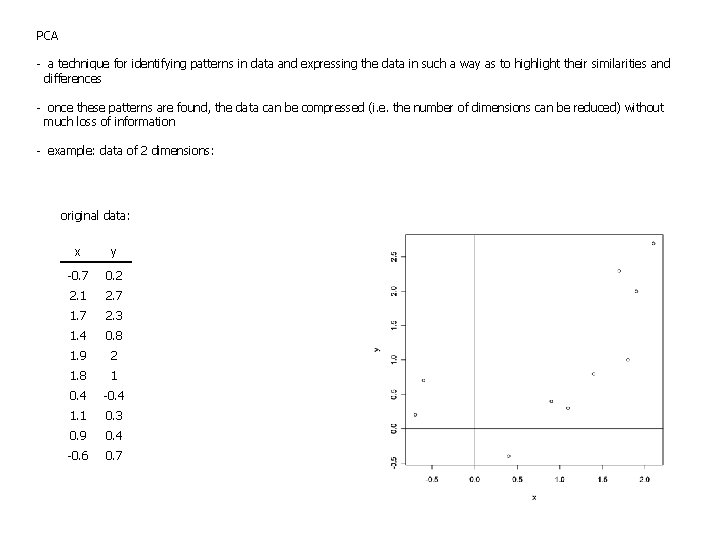PCA - a technique for identifying patterns in data and expressing the data in such a way as to highlight their similarities and differences - once these patterns are found, the data can be compressed (i. e. the number of dimensions can be reduced) without much loss of information - example: data of 2 dimensions: original data: x y -0. 7 0. 2 2. 1 2. 7 1. 7 2. 3 1. 4 0. 8 1. 9 2 1. 8 1 0. 4 -0. 4 1. 1 0. 3 0. 9 0. 4 -0. 6 0. 7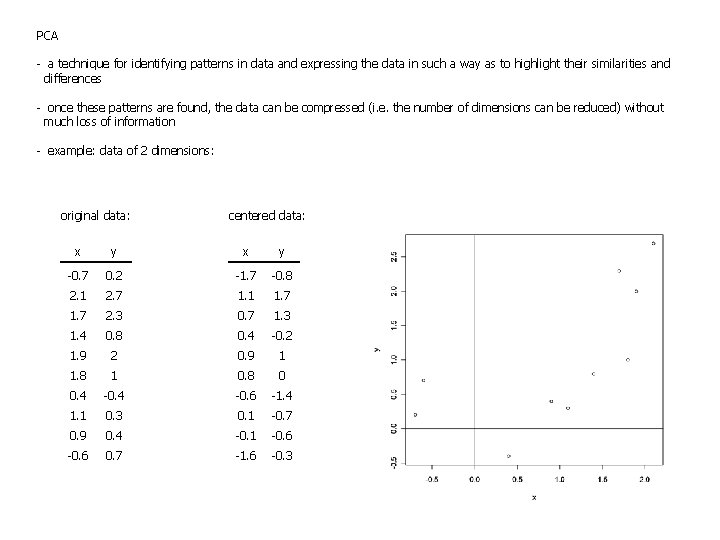PCA - a technique for identifying patterns in data and expressing the data in such a way as to highlight their similarities and differences - once these patterns are found, the data can be compressed (i. e. the number of dimensions can be reduced) without much loss of information - example: data of 2 dimensions: original data: centered data: x y -0. 7 0. 2 -1. 7 -0. 8 2. 1 2. 7 1. 1 1. 7 2. 3 0. 7 1. 3 1. 4 0. 8 0. 4 -0. 2 1. 9 2 0. 9 1 1. 8 1 0. 8 0 0. 4 -0. 6 -1. 4 1. 1 0. 3 0. 1 -0. 7 0. 9 0. 4 -0. 1 -0. 6 0. 7 -1. 6 -0. 3PCA - a technique for identifying patterns in data and expressing the data in such a way as to highlight their similarities and differences - once these patterns are found, the data can be compressed (i. e. the number of dimensions can be reduced) without much loss of information - example: data of 2 dimensions: original data: centered data: x y -0. 7 0. 2 -1. 7 -0. 8 2. 1 2. 7 1. 1 1. 7 2. 3 0. 7 1. 3 1. 4 0. 8 0. 4 -0. 2 1. 9 2 0. 9 1 1. 8 1 0. 8 0 0. 4 -0. 6 -1. 4 1. 1 0. 3 0. 1 -0. 7 0. 9 0. 4 -0. 1 -0. 6 0. 7 -1. 6 -0. 3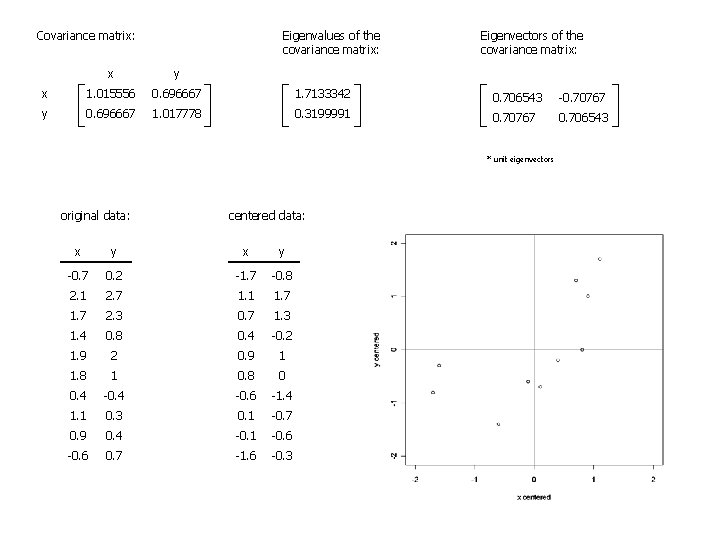Eigenvalues of the covariance matrix: Covariance matrix: Eigenvectors of the covariance matrix: x y x 1. 015556 0. 696667 1. 7133342 0. 706543 -0. 70767 y 0. 696667 1. 017778 0. 3199991 0. 70767 0. 706543 * unit eigenvectors original data: centered data: x y -0. 7 0. 2 -1. 7 -0. 8 2. 1 2. 7 1. 1 1. 7 2. 3 0. 7 1. 3 1. 4 0. 8 0. 4 -0. 2 1. 9 2 0. 9 1 1. 8 1 0. 8 0 0. 4 -0. 6 -1. 4 1. 1 0. 3 0. 1 -0. 7 0. 9 0. 4 -0. 1 -0. 6 0. 7 -1. 6 -0. 3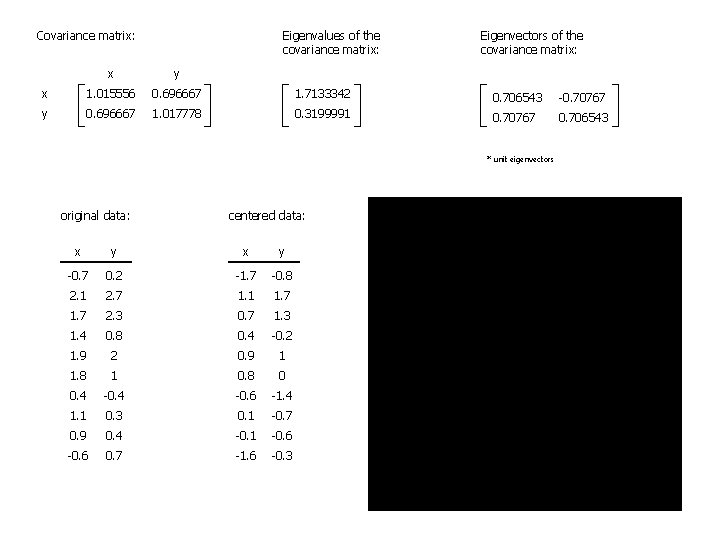Eigenvalues of the covariance matrix: Covariance matrix: Eigenvectors of the covariance matrix: x y x 1. 015556 0. 696667 1. 7133342 0. 706543 -0. 70767 y 0. 696667 1. 017778 0. 3199991 0. 70767 0. 706543 * unit eigenvectors original data: centered data: x y -0. 7 0. 2 -1. 7 -0. 8 2. 1 2. 7 1. 1 1. 7 2. 3 0. 7 1. 3 1. 4 0. 8 0. 4 -0. 2 1. 9 2 0. 9 1 1. 8 1 0. 8 0 0. 4 -0. 6 -1. 4 1. 1 0. 3 0. 1 -0. 7 0. 9 0. 4 -0. 1 -0. 6 0. 7 -1. 6 -0. 3Compression and reduced dimensionality: - the eigenvector associated with the highest eigenvalue is the principal component of the dataset; it captures the most significant relationship between the data dimensions - ordering eigenvalues from highest to lowest gives the components in order of significance - if we want, we can ignore the components of lesser significance – this results in a loss of information, but if the eigenvalues are small, the loss will not be great - thus, in a dataset with n dimensions/variables, one may obtain the n eigenvectors & eigenvalues and decide to retain p of them → this results in a final dataset with only p dimensions - feature vector – a vector containing only the eigenvectors representing the dimensions we want to keep – in our example data, 2 choices: 0. 706543 -0. 70767 0. 706543 or 0. 706543 0. 70767 - the final dataset is obtained by multiplying the transpose of the feature vector (on the left) with the transposed original dataset - this will give us the original data solely in terms of the vectors we chose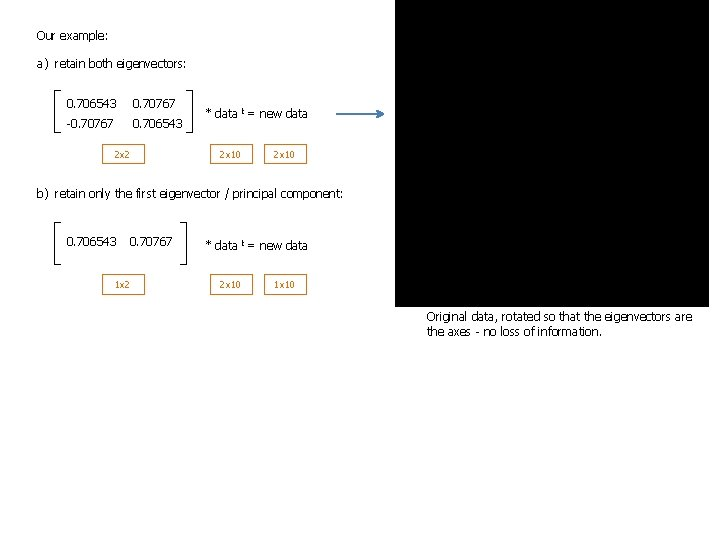Our example: a) retain both eigenvectors: 0. 706543 0. 70767 -0. 70767 0. 706543 2 x 2 * data t = new data 2 x 10 b) retain only the first eigenvector / principal component: 0. 706543 0. 70767 1 x 2 * data t = new data 2 x 10 1 x 10 Original data, rotated so that the eigenvectors are the axes - no loss of information.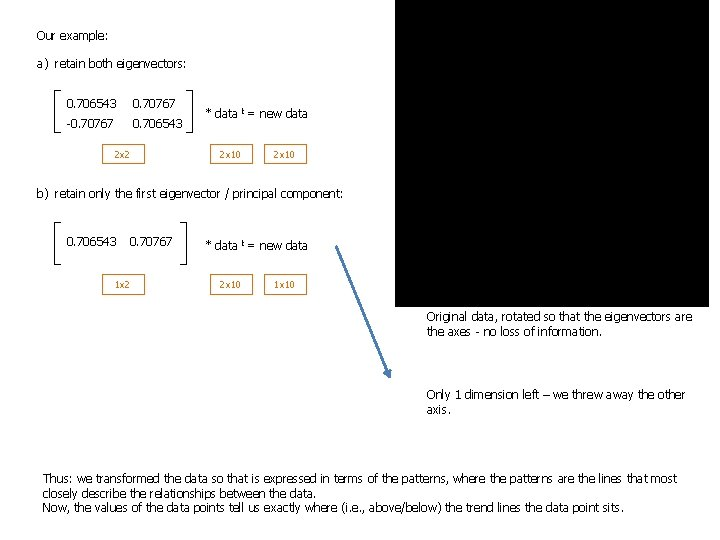Our example: a) retain both eigenvectors: 0. 706543 0. 70767 -0. 70767 0. 706543 2 x 2 * data t = new data 2 x 10 b) retain only the first eigenvector / principal component: 0. 706543 0. 70767 1 x 2 * data t = new data 2 x 10 1 x 10 Original data, rotated so that the eigenvectors are the axes - no loss of information. Only 1 dimension left – we threw away the other axis. Thus: we transformed the data so that is expressed in terms of the patterns, where the patterns are the lines that most closely describe the relationships between the data. Now, the values of the data points tell us exactly where (i. e. , above/below) the trend lines the data point sits.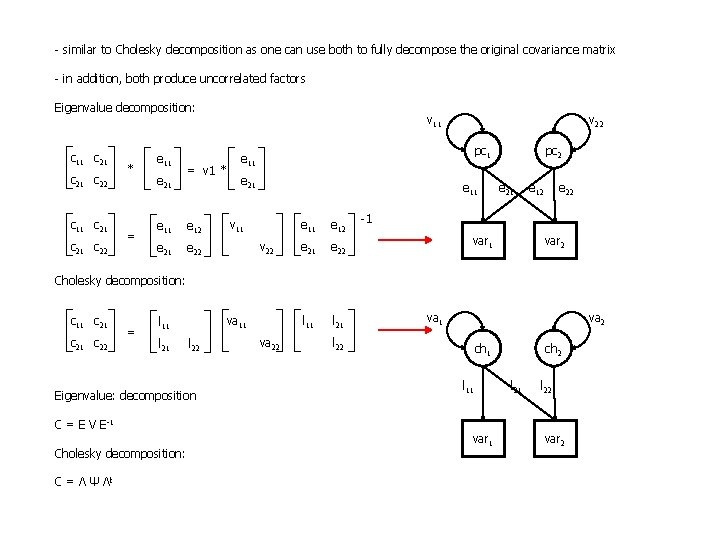- similar to Cholesky decomposition as one can use both to fully decompose the original covariance matrix - in addition, both produce uncorrelated factors Eigenvalue decomposition: c 11 c 21 c 22 * = e 11 e 21 = v 1 * e 11 e 12 e 21 e 22 v 11 v 22 pc 1 e 11 e 21 e 11 v 22 e 11 e 12 e 21 e 22 l 11 l 21 pc 2 e 21 e 12 e 22 -1 var 2 Cholesky decomposition: c 11 c 21 c 22 = va 11 l 21 l 22 Eigenvalue: decomposition C = E V E-1 Cholesky decomposition: C = Λ Ψ Λt va 22 l 22 va 1 va 2 ch 1 l 11 var 1 ch 2 l 21 l 22 var 2